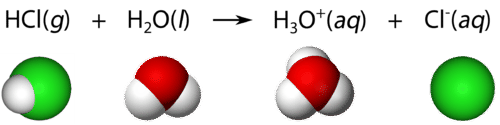# 5.14: Arrhenius Acids

$$\newcommand{\vecs}{\overset { \rightharpoonup} {\mathbf{#1}} }$$ $$\newcommand{\vecd}{\overset{-\!-\!\rightharpoonup}{\vphantom{a}\smash {#1}}}$$$$\newcommand{\id}{\mathrm{id}}$$ $$\newcommand{\Span}{\mathrm{span}}$$ $$\newcommand{\kernel}{\mathrm{null}\,}$$ $$\newcommand{\range}{\mathrm{range}\,}$$ $$\newcommand{\RealPart}{\mathrm{Re}}$$ $$\newcommand{\ImaginaryPart}{\mathrm{Im}}$$ $$\newcommand{\Argument}{\mathrm{Arg}}$$ $$\newcommand{\norm}{\| #1 \|}$$ $$\newcommand{\inner}{\langle #1, #2 \rangle}$$ $$\newcommand{\Span}{\mathrm{span}}$$ $$\newcommand{\id}{\mathrm{id}}$$ $$\newcommand{\Span}{\mathrm{span}}$$ $$\newcommand{\kernel}{\mathrm{null}\,}$$ $$\newcommand{\range}{\mathrm{range}\,}$$ $$\newcommand{\RealPart}{\mathrm{Re}}$$ $$\newcommand{\ImaginaryPart}{\mathrm{Im}}$$ $$\newcommand{\Argument}{\mathrm{Arg}}$$ $$\newcommand{\norm}{\| #1 \|}$$ $$\newcommand{\inner}{\langle #1, #2 \rangle}$$ $$\newcommand{\Span}{\mathrm{span}}$$$$\newcommand{\AA}{\unicode[.8,0]{x212B}}$$

Venus is the planet nearest to Earth, but has a very different and hostile environment. It has a surface temperature that averages around $$450^\text{o} \text{C}$$. The atmosphere is composed of carbon dioxide, but clouds of sulfuric acid move through the upper atmosphere, helping to create the extremely unfriendly conditions. Because of these conditions, Venus is not a place you want to visit on vacation.

## Arrhenius Acids

Swedish chemist Svante Arrhenius (1859-1927) was the first to propose a theory to explain the observed behavior of acids and bases. Because of their ability to conduct a current, he knew that both acids and bases contained ions in solution. An Arrhenius acid is a compound that ionizes to yield hydrogen ions $$\left( \ce{H^+} \right)$$ in aqueous solution.

Acids are molecular compounds with ionizable hydrogen atoms. Only hydrogen atoms that are part of a highly polar covalent bond are ionizable. Hydrogen chloride $$\left( \ce{HCl} \right)$$ is a gas at room temperature and under normal pressure. The $$\ce{H-Cl}$$ bond in hydrogen chloride is a polar bond. The hydrogen atom is electron deficient because of the higher electronegativity of the chlorine atom. Consequently, the hydrogen atom is attracted to the lone pair of electrons in a water molecule when $$\ce{HCl}$$ is dissolved in water. The result is that the $$\ce{H-Cl}$$ bond breaks, with both bonding electrons remaining with the $$\ce{Cl}$$, forming a chloride ion. The $$\ce{H^+}$$ ion attaches to the water molecule, forming a polyatomic ion called the hydronium ion. The hydronium ion $$\left( \ce{H_3O^+} \right)$$ can be thought of as a water molecule with an extra attached hydrogen ion.Figure $$\PageIndex{1}$$: Formation of a hydronium ion. (CC BY-NC; CK-12)

Equations showing the ionization of an acid in water are frequently simplified by omitting the water molecule:

$\ce{HCl} \left( g \right) \rightarrow \ce{H^+} \left( aq \right) + \ce{Cl^-} \left( aq \right)\nonumber$

This is merely a simplification of the previous equation, but it is commonly used. Any hydrogen ions in an aqueous solution will be attached to water molecules as hydronium ions.

Not all hydrogen atoms in molecular compounds are ionizable. In methane $$\left( \ce{CH_4} \right)$$, the hydrogen atoms are covalently bonded to carbon in bonds that are only slightly polar. The hydrogen atoms are not capable of ionizing, and methane has no acidic properties. Acetic acid $$\left( \ce{CH_3COOH} \right)$$ belongs to a class of acids called organic acids. There are four hydrogen atoms in the molecule, but only the one hydrogen that is bonded to an oxygen atom is ionizable.Figure $$\PageIndex{2}$$: The $$\ce{O-H}$$ bond can be ionized to yield the $$\ce{H^+}$$ ion and the acetate ion. The other hydrogen atoms in this molecule are not acidic. (CC BY-NC; CK-12)

The table below lists some of the more common acids:

Common Acids
Table $$\PageIndex{1}$$: Common Acids
Acid Name Formula
Hydrochloric acid $$\ce{HCl}$$
Nitric acid $$\ce{HNO_3}$$
Sulfuric acid $$\ce{H_2SO_4}$$
Phosphoric acid $$\ce{H_3PO_4}$$
Acetic acid $$\ce{CH_3COOH}$$
Hypochlorous acid $$\ce{HClO}$$

A monoprotic acid is an acid that contains only one ionizable hydrogen. Hydrochloric acid and acetic acid are monoprotic acids. A polyprotic acid is an acid that contains multiple ionizable hydrogens. Most common polyprotic acids are either diprotic (such as $$\ce{H_2SO_4}$$) or triprotic (such as $$\ce{H_3PO_4}$$).

## Summary

• An Arrhenius acid is a compound that ionizes to yield hydrogen ions $$\left( \ce{H^+} \right)$$ in aqueous solution.
• Examples of Arrhenius acids are given.

5.14: Arrhenius Acids is shared under a CC BY-NC license and was authored, remixed, and/or curated by LibreTexts.